Courses

# Ecology And Evolution - (EY) 2018 GATE Paper (Practice Test)

## 65 Questions MCQ Test GATE Past Year Papers for Practice (All Branches) | Ecology And Evolution - (EY) 2018 GATE Paper (Practice Test)

Description
This mock test of Ecology And Evolution - (EY) 2018 GATE Paper (Practice Test) for GATE helps you for every GATE entrance exam. This contains 65 Multiple Choice Questions for GATE Ecology And Evolution - (EY) 2018 GATE Paper (Practice Test) (mcq) to study with solutions a complete question bank. The solved questions answers in this Ecology And Evolution - (EY) 2018 GATE Paper (Practice Test) quiz give you a good mix of easy questions and tough questions. GATE students definitely take this Ecology And Evolution - (EY) 2018 GATE Paper (Practice Test) exercise for a better result in the exam. You can find other Ecology And Evolution - (EY) 2018 GATE Paper (Practice Test) extra questions, long questions & short questions for GATE on EduRev as well by searching above.
QUESTION: 1

Solution:
QUESTION: 2

Solution:
QUESTION: 3

### Seven machines take 7 minutes to make 7 identical toys. At the same rate, how many minutes would it take for 100 machines to make 100 toys?

Solution:
QUESTION: 4

A rectangle becomes a square when its length and breadth are reduced by 10 m and 5 m, respectively. During this process, the rectangle loses 650 m2 of area. What is the area of the original rectangle in square meters?

Solution:
QUESTION: 5

A number consists of two digits. The sum of the digits is 9. If 45 is subtracted from the number, its digits are interchanged. What is the number?

Solution:
QUESTION: 6

For integers a, b and c, what would be the minimum and maximum values respectively of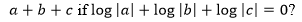Solution:
QUESTION: 7

Given that a and b are integers and a + a2 b3 is odd, which one of the following statements is correct?

Solution:
QUESTION: 8

From the time the front of a train enters a platform, it takes 25 seconds for the back of the train to leave the platform, while travelling at a constant speed of 54 km/h. At the same speed, it takes 14 seconds to pass a man running at 9 km/h in the same direction as the train. What is the length of the train and that of the platform in meters, respectively?

Solution:
QUESTION: 9

Which of the following functions describe the graph shown in the below figure?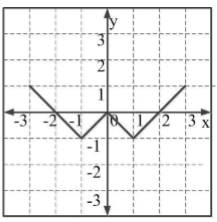Solution:
QUESTION: 10

Consider the following three statements:
(i) Some roses are red.
(ii) All red flowers fade quickly.
(iii) Some roses fade quickly.
Which of the following statements can be logically inferred from the above statements?

Solution:
QUESTION: 11

During the Pleistocene, which of the following groups experienced the largest mass extinction?

Solution:
QUESTION: 12

In group living species, the term “dilution effect” refers to

Solution:
QUESTION: 13

Which of the following diversity indices best captures species turnover across habitats?

Solution:
QUESTION: 14

A duck egg is removed from its mother’s nest and incubated by a barnyard hen. The duckling hatches out in the presence of the barnyard hen and stays in her nest. After a couple of days, the duckling is presented with a choice between its biological mother and the hen that incubated it. The duckling approaches and follows the hen. This set of observations demonstrates the phenomenon of

Solution:
QUESTION: 15

If both of your ears were located next to each other in the middle of your face, you would have difficulty in resolving the

Solution:
QUESTION: 16

Forager bees communicate the distance of a food source using a waggle dance upon return to the hive. It is observed that the number of waggles of a dance increases linearly with the distance to the food source. This tells us that the

Solution:
QUESTION: 17

Male frogs display to females by producing loud acoustic signals. The use of these signals by bats to locate frogs and prey upon them is an example of

Solution:
QUESTION: 18

A non-venomous, non-toxic species of snake is brightly coloured and closely resembles a venomous snake species in the same habitat. This is most likely a case of

Solution:
QUESTION: 19

The value of the resting membrane potential of a typical neuron is closest to the equilibrium potential of which of the following ions?

Solution:
QUESTION: 20

A plant species found in India produces flowers that are white, fragrant, and tubular. Which of the following is the most likely pollinating agent?

Solution:
QUESTION: 21

Which of the following can be used to test differences between mean tree heights in a tropical versus a temperate forest?

Solution:
QUESTION: 22

For a species, assuming a relatively short time scale and no evolution, increased interspecific competition will result in a

Solution:
QUESTION: 23

In which of the following mating systems is sperm competition likely to evolve?

Solution:
QUESTION: 24

Copies of genes that arrive in a particular genome by horizontal gene transfer are known as

Solution:
QUESTION: 25

In which of the following plants is the dominant stage of the life cycle haploid?

Solution:
QUESTION: 26

Which of the following is NOT typically a characteristic of early successional pioneer plants relative to late successional plants in a tropical rain forest?

Solution:
QUESTION: 27

Natural populations often deviate from Hardy-Weinberg equilibrium. One possible reason for this is

Solution:
QUESTION: 28

Which of the following is NOT an example of phenotypic plasticity?

Solution:
QUESTION: 29

Which of the following is NOT a characteristic of r-selected species?

Solution:
QUESTION: 30

A scientist finds a new species of insect and sends a sample to a museum for confirmation. The curator of the museum designates it as a holotype for the newly identified species and asks the scientist to also provide samples of the opposite sex. This sample of the opposite sex is called the

Solution:
QUESTION: 31

Which of the following is NOT essential for a behavioural trait to evolve by natural selection?

Solution:
QUESTION: 32

El Niño Southern Oscillation (ENSO) events have occurred approximately every 3-7 years over the last century. The cause of these ENSO events is related to

Solution:
QUESTION: 33

If the mean of a sample is 5, and the variance is 25, the PERCENT coefficient of variation is ___.

Solution:
QUESTION: 34

Consider a diploid population at Hardy-Weinberg equilibrium. For a locus with two alleles, the frequency of the A1A1 genotype is 0.01. The frequency of heterozygotes A1A2 is ____ (answer up to 2 decimal places).

Solution:
QUESTION: 35

In a food chain, the efficiency of transfer of energy from one trophic level to the next is 10%. The PERCENTAGE of energy that is expected to transfer from the second to the fourth level is ____.

Solution:
QUESTION: 36

Which of the following is an adaptation for osmoregulation in freshwater teleost fish?

Solution:
QUESTION: 37

The latitudinal diversity gradient is defined as the decrease in the number of species from the equator to the poles. This can result from

Solution:
QUESTION: 38

Identify the graph in which natural selection on beak size is LEAST likely to be occurring? For each of the graphs, the slope of the regression line and the associated p-value is given.

Solution:
QUESTION: 39

Some species of spiders add additional silk ‘decorations’ to their webs. It is hypothesized that these decorations serve either to lure flies (prey) or to decrease bird (predator) attacks on the spiders. The appropriate way to test these hypotheses would be to compare numbers of

Solution:
QUESTION: 40

The figure below shows mean annual temperatures (°C) and mean annual precipitation (cm per year) from multiple sites in four regions in India.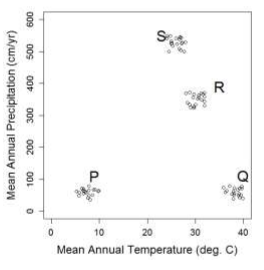From this climatic information, we can infer that P, Q, R, and S are LIKELY to be located in:

Solution:
QUESTION: 41

For a pair of interacting species, increased specialization and decreased niche breadth in both species would result in

Solution:
QUESTION: 42

Males of a bird species provide parental care by feeding nestlings before they fledge. Which of the following is a PROXIMATE explanation for this behaviour?

Solution:
QUESTION: 43

The courtship display of a spider species consists of both visual and vibrational signals. Females respond to the display by approaching males. In an experiment, females were presented with 3 treatments: i) only videos of displaying males, ii) only vibrational signals of the display, and iii) both videos of displaying males and vibrational signals. The results are given below: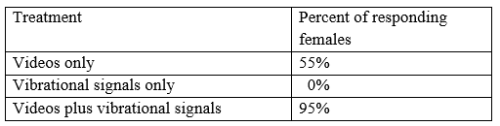Results from this experiment show that

Solution:
QUESTION: 44

Moth ears typically consist of a membranous eardrum backed by an air cavity. Ears in different phylogenetic groups of moths have evolved on different body parts. Moth ears are thus best described as

Solution:
QUESTION: 45

Increased anthropogenic disturbance has resulted in an overall decrease in densities of trees and an increase in fragmentation of forests. Which of the following types of trees will have the greatest reduction in reproductive success?

Solution:
QUESTION: 46

A study examined the effect of neighbours on plants when grown at low or high altitudes. The researcher measured Relative Neighbour Effect (RNE), defined as: RNE = Biomass with neighbours – Biomass without neighbours.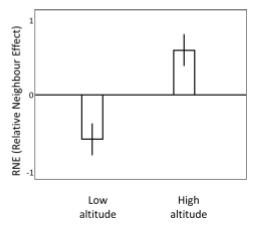The results shown above demonstrate

Solution:
QUESTION: 47

Match the combination of flora and fauna from the list, to the state where they are found.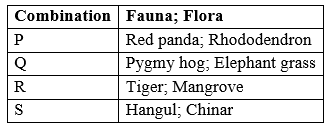Solution:
QUESTION: 48

A parent population (P) is split into three daughter populations (Q, R, and S) which grow in three different habitats. After 1000 generations, the equilibrium frequency distribution of a trait in each of the daughter populations is shown in the figure below. For reference, the vertical line represents the mean of the parent population.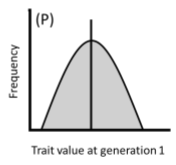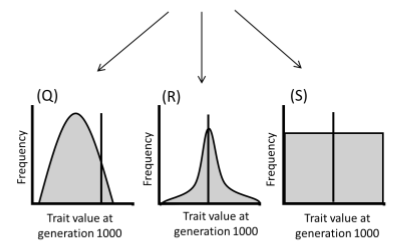From this information, one can infer that the subpopulations experienced the following selection regimes

Solution:
QUESTION: 49

The character matrix below lists four taxa (P-S) and their nine characters (i-ix). A character state is designated as ‘0’ if it is ancestral and ‘1’ if it is derived. Which of the following phylogenetic trees is obtained by cladistic analysis of these data?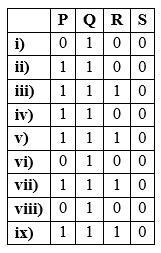Solution:
QUESTION: 50

The reactions below (R1 and R2) represent different carbon fixation pathways in photosynthesis.
RuBP represents Ribulose bisphosphate.
Rubisco represents RuBP carboxylase-oxygenase.
PGA represents Phosphoglyceric acid.
PEP represents Phosphoenolpyruvate.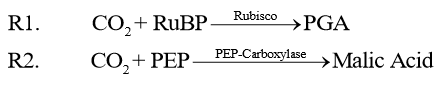Which of the following is CORRECT?

Solution:
QUESTION: 51

A fish species is sexually dimorphic: males possess ultraviolet (UV) spots on their bodies which are lacking in females. Females prefer males with larger and more intense UV spots as mates. Which of the following statements is a plausible reason for the spots being coloured ultraviolet?

Solution:

A (or) D

QUESTION: 52

A climate scientist notices a trend in atmospheric CO2 concentrations (in ppm) at a research station. Although overall CO2 is rising over decades, there are intra-annual fluctuations, as shown in the figure below.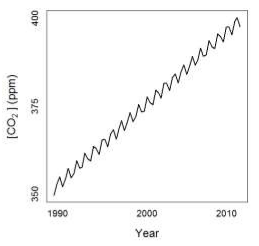These fluctuations can be attributed to

Solution:
QUESTION: 53

Four islands differ in size (small = 10 km2, large = 100 km2) and distance from the mainland (near = 50 km, far = 500 km).
Island P is small and near the mainland;
Island Q is small and far from the mainland;
Island R is large and near the mainland;
Island S is large and far from the mainland
Let NP, NQ, NR and NS denote the number of species on islands P, Q, R and S, respectively. Which of the following is consistent with the theory of island biogeography?

Solution:
QUESTION: 54

The rate of population growth (dN/dt) over time (t) is shown below.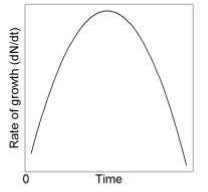For the above population, which of the following plots of population (Popn.) size (N) vs. time (t) is CORRECT?

Solution:
QUESTION: 55

The pedigree below details a late onset genetic disease among humans. Males are represented as squares and females as circles. Individuals with the disease are depicted as black, and those without it are depicted as white. Which of the following best describes the pattern of inheritance of the disease-causing gene?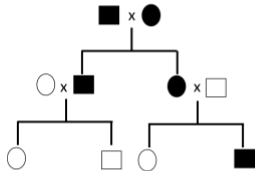Solution:
QUESTION: 56

Match the following scientists with the concepts or theories they are associated with.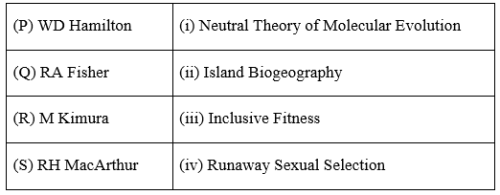Solution:
QUESTION: 57

Which of the following is an example of complete intrinsic post-zygotic reproductive isolation between two species P and Q?

Solution:
QUESTION: 58

Match the animals to their locomotor adaptation.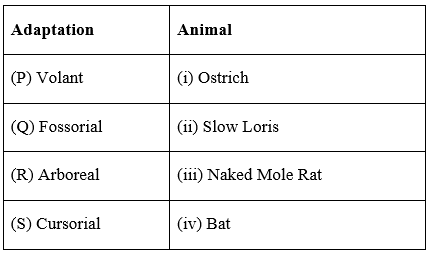Solution:
QUESTION: 59

Paralogs are genes that are the products of gene duplication events within a species. Orthologs are genes in different species that share a common ancestral gene. The figure below describes the evolutionary history of a hypothetical gene X in organism Y. This gene undergoes a duplication event. Later, Y splits into two species Y1 and Y2, and this gives rise to four copies of the gene - P, Q, R and S. Which option best describes the relationships between the four copies of the gene X?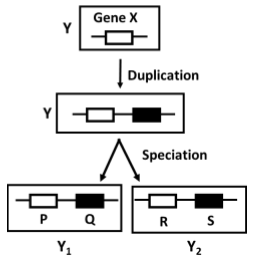Solution:
QUESTION: 60

The frequency distribution of beak sizes of a bird species is symmetric but not normally distributed. If the mean value of beak size is 6 mm, standard deviation is 25 mm and kurtosis is 10, then the median is ____ mm.

Solution:
QUESTION: 61

An altruist provides help worth 10 units of fitness to a recipient, at a personal cost of 1 unit of fitness. As per kin-selection theory, the minimum value of genetic relatedness between the actors and the recipients that is necessary to maintain altruism in the population is _____ (answer up to 1 decimal place).

Solution:
QUESTION: 62

A population grows from a size of 100 individuals at t = 0, to 1000 individuals at t = 100, following density-independent growth. The ratio of per-capita growth rates at the initial (at t = 0) to the final (t = 100) time is ____.

Solution:
QUESTION: 63

The probability that a bush has a cricket is 0.1. The probability of a spider being present on a bush is 0.2. When both a spider and a cricket are present on a bush, the probability of encountering each other is 0.2. The probability of a spider consuming a cricket it encounters is 0.5. Assuming that predation only occurs on bushes, the probability that a cricket is preyed on by a spider is ____ (answer up to 3 decimal places).

Solution:
QUESTION: 64

A plant produces seeds that can be dispersed by birds or mammals. The probability that a seed is dispersed by a bird is 0.25, and by a mammal is 0.5. The bird can disperse a seed to three patches A, B, or C with a probability 0.5, 0.4 or 0.1, respectively.  On the other hand, the mammal disperses a seed to the same patches A, B, or C, with a probability 0.15, 0.8 and 0.05, respectively. The probability that a given seed is dispersed to patch B is ___ (answer up to 1 decimal place).

Solution:
QUESTION: 65

The species area relationship of trees in Mudumalai Tiger Reserve is given by S = 0.1 × A0.3, where S is the number of species in a given area A. When log S is plotted against log A, the slope of the resulting relationship is _____ (answer up to 1 decimal place).

Solution: